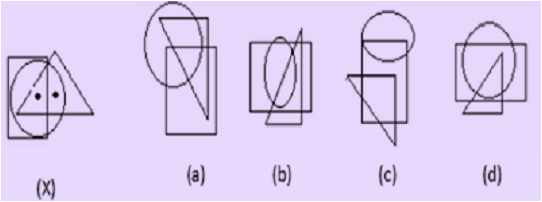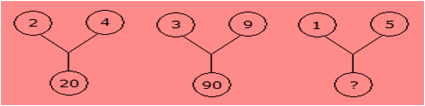# Indian Talent Olympiad - IMO PDF Sample Papers for Class 8

Class 8 sample paper & practice questions for International Maths Olympiad (IMO) level 1 are given below. Syllabus for level 1 is also mentioned for these exams. You can refer these sample paper & quiz for preparing for the exam.#### Resources:

##### Sample Questions from Olympiad Success:
 Q.1 Q.2 Q.3 Q.4 Q.5 Q.6 Q.7 Q.8 Q.9 Q.10
 Q.1 Simple interest on a certain sum is 16/25 of the sum. Find the rate percent and the time if both are numerically equal. a) 64% and 64 b) 8% and 8 c) 12% and 12 d) 8.2% and 8.2
 Q.2 Expansion of -8(3a + 5b) yields: a) -24a - 40b b) -22a + 80b c) 20a - 30b d) -24a - 41b
 Q.3 Which of the following statements is always true: a) A square number cannot be the sum of two other square numbers b) A number that has 5 in the units place can never be a perfect square c) A number that leaves a remainder of 3 when divided by 4 cannot be a perfect square d) A number that has 7 in the units place may be a perfect square
 Q.4 Find the value of the expression 2x3 - x2 - 3, when x = 3. a) 66 b) 52 c) 32 d) 42
 Q.5 How many non-square numbers lie between 112 and 122? a) 9 b) 10 c) 8 d) 11
 Q.6 Which of the following is not the property of a rectangle? a) a rectangle is a parallelogram in which one of  the angles is a right angle b) diagonals of a rectangle are equal c) opposite sides of a rectangle are equal d) diagonals of a rectangle bisect each other at right angle
 Q.7 Select the figure which satisfies the same conditions of placement of the dots as in fig. (X).a) a b) b c) c d) d
 Q.8 Which of the following is a factor of xy + x - y -1? a) x + 1 b) y + 1 c) x + y d) x - y
 Q.9 Find the missing character from among the given alternatives:a) 75 b) 20 c) 25 d) 26
 Q.10 Whole number such that twice of its square added to itself gives 10? a) 5 b) 2 c) 8 d) 10Sample PDF of Indian Talent Olympiad - International Maths Olympiad (IMO) PDF Sample Papers for Class 8:

 Q.1 )b Q.2 )a Q.3 )c Q.4 )d Q.5 )c Q.6 )d Q.7 )a Q.8 )b Q.9 )d Q.10 )b

Q.1 : b | Q.2 : a | Q.3 : c | Q.4 : d | Q.5 : c | Q.6 : d | Q.7 : a | Q.8 : b | Q.9 : d | Q.10 : b